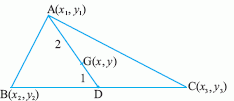Email us to get an instant 20% discount on highly effective K-12 Math & English kwizNET Programs!

#### Online Quiz (WorksheetABCD)

Questions Per Quiz = 2 4 6 8 10

### High School Mathematics - 29.16 Centroid of a Triangle

 To find the centroid of a triangle whose vertices are givenLet A(x1, y1), B(x2, y2) and C(x3, y3) be the vertices of the triangle ABC. Let AD be the median bisecting the base. Then D = {(x2 + x3)/2, (y2 + y3)/2} Now the point G on AD, which divides it internally in the ratio 2:1, is the centroid. If (x,y) are the coordinates of G, then x = 2x(x2+x3)/2 + (1 x x1)/2+1 = (x1+x2+x3)/3 y = 2x(y2+y3)/2 + (1 x y1)/(2+1) = (y1+y2+y3)/3 Hence, the coordinates of the centroid are given by x = (x1+x2+x3)/3, y = (y1+y2+y3)/3. Directions: Solve the following.
 Q 1: The vertices of a triangle are (1,2), (h, -3) and (-4, k). FInd the values of h and k if the centroid of the triangle be at the point (5, -1).(2, 18)(-18, -2)(18, -2)(8, 2) Q 2: Find the centroid of the triangle whose angular points are (-1,0), (5, -2) and (8, -2)(-4,0)(0, 4)(4, 0)(0, -4) Q 3: Find the third vertex of a triangle if two of its vertices are at (-1,4) and (5,2)(-4, -15)(4, -15)(4, 15)(-4, 15) Q 4: Find the centroid of the triangle whose sides are formed by the lines 2x-3y = 1, y + 1 = 0 and 4x - 5y = 1.(1/3, 1/3)(0,0)(1/3, 0)(0, 1/3) Q 5: Origin is the centroid of the triangle, whose two vertices are (5, -1), (-2,3).Find the third vertex.(3,-2)(-3, -2)(3, 2) Q 6: Find the centroid of the triangle formed by the lines 3x-y-11 = 0, 7y+x-11 = 0, 2x+3y = 0(4/3, 1/3)(-4/3, 1/3)(-4/3, -1/3) Q 7: Find the length of the median AD of a triangle whose vertices are A(4,4), B(3,-2) and C(-3,16).37105 Q 8: The coordnates of the vertices of the triangle are (4, -3), (-5,2) and (x,y). If the centroid of the triangle is at the origin, what is the value of x and y.01(0,1)(1,1) Question 9: This question is available to subscribers only! Question 10: This question is available to subscribers only!

#### Subscription to kwizNET Learning System offers the following benefits:

• Unrestricted access to grade appropriate lessons, quizzes, & printable worksheets
• Instant scoring of online quizzes
• Progress tracking and award certificates to keep your student motivated
• Unlimited practice with auto-generated 'WIZ MATH' quizzes